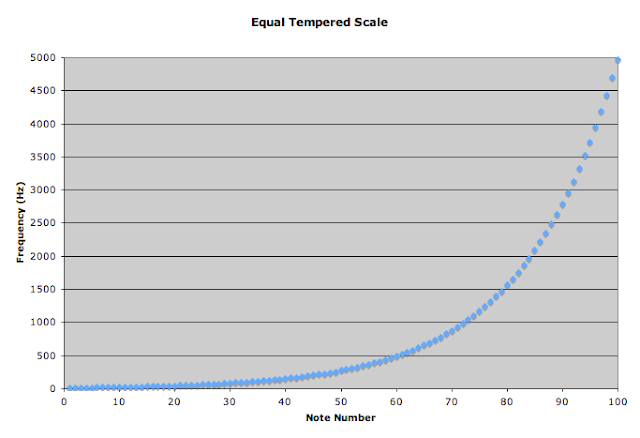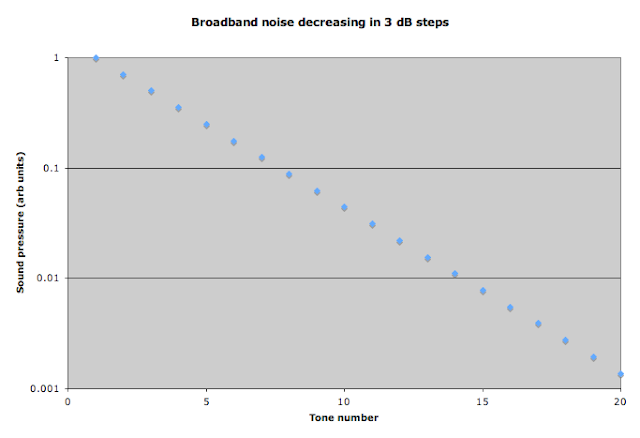## August 31, 2011

### Logarithmic scaling in acoustics

I want to say first that I really like what Vi Hart does with her videos.

Last week a few people in my twitter stream linked to a pair of videos that Vi did with Sal Khan of the Khan Academy.  Here's one of them:

The point of this video (as I see it) is to discuss the difference between linear and logarithmic scales. It's a great concept that deserves discussion, and they make a decent effort to get their point across, I think.

It's just that the examples from the musical scales are filled with little inaccuracies that could easily have been corrected before they recorded the video.

Vi says at one point (somewhere around the 3:50 mark and after) "C is more like ...I don't know...let's say 300 all right so if this is 300 or 300x or just x...then this frequency would be 600..." Sal was trying to help her out since she didn't know the note frequencies that she was trying to use in her example, so he suggested just calling it "x". I think there can be more confusion introduced by trying to use the "x", but maybe that's just me.   I can get past that, I guess.

There is a whole lot wrong with this small section, I barely know where to start. First, there are no units included at all. Actually, Sal is trying to help Vi by suggesting that they use 440 as a note frequency, but he actually starts to say 440 kHz.  The highest frequency the human ear can hear is around the 20 kHz range for healthy (and young) people. So hundreds of kHz are way out of the range of hearing.  But, Vi wants to use C and picks the number 300 to work with, no units included. This is something I don't let freshman get away with on their work, and I work hard to not let myself forget units, either.

Okay, though, we'll assume the units they are using are Hz. Why pick 300 Hz for a C? (Clearly, she forgot to look up note frequencies. I get it.) Next time Sal and Vi talk about musical notes, I'm sure they will have the note frequencies handy. Why is this important? There is an international standard for note frequencies. Without making this all about tuning and temperament and the origin of harmony, let me say that the standard says that the pitch we call A above middle C is associated with 440 Hz. Based on that standard, if we are looking at an equal temperament scale (the most commonly used scale in music), the frequency of middle C is then 261.63 Hz.  Vi even comments that they have chosen a really "weird" musical scale. Yeah, no kidding.

I know that her point was that the difference in frequencies between notes separated by an octave increases as you go up the keyboard, but is it so hard to use the right frequencies? If the point of posting videos is to be educational, then why have wrong information in them? The details matter. It reminds me of the tuning fork set which have C labeled as having a frequency in multiples of 256 Hz. There have been proposed scales which are based around middle C having a frequency of 256 Hz, but no musician uses this scale today.  Yet, we have countless physics and math teachers who believe that middle C on a piano has a fundamental frequency of 256 Hz. It's unfortunate, because the teachers and their students are missing an opportunity to learn more about music when they use this artificial scientific scale.

Here's a graph of the note frequencies for the equal tempered scale which I took from the page linked above. The horizontal axis is arbitrarily labeled "note number". The point of the graph is to show how the frequency difference between each successive note changes over a wide range of octaves. Each successive point on the graph represents a semitone higher in pitch than the previous note. Your ear perceives each tone as being the same "distance" in pitch as the previous tone, even though the change in frequency is not the same over the whole range.Let's take the same graph, and make a semi-log plot.  Here, I'm making the vertical axis (frequency) logarithmic.Notice how now it is a straight line? This is what it means for something to scale logarithmically. The wikipedia page for logarithmic scale has some more examples.

Also, it was too bad that they didn't have any of the actual tones in the examples they were using.  Here's a piano keyboard you can use to hear for yourself how the intervals sound similar across octaves:

ButtonBeats.com

Toward the end of the video (around the 6:00 minute mark) Sal makes a comment that the logarithmic scale of frequencies is not the only logarithmic aspect to sound. He refers to the "magnitude of frequencies" and then quickly amends his statement to refer to the decibel scale. Vi chimes in with an example of talking loudly and softly, then starts to say something about the "distances between loudnesses" before Sal interrupts and the video wraps up.  I have no idea what "magnitude of frequencies" and "distances between loudnesses" actually mean.  From the context, it's clear that they are talking about the sound pressure, or sound intensity, or even sound power. All of those are ways to quantify the amplitude of a sound wave. They all mean different things, but the relevant point is that they all scale logarithmically, in a way similar to the pitch scale discussed above.

It's too bad they didn't take the time to go into the example of sound intensity and the decibel level. It's a great example of logarithmic scaling.  Here's an example from an awesome website on musical acoustics at the University of New South Wales in Australia:

Credit for the sound files and flash animation: John Tann and George Hatsidimitris

The example has broadband noise decreasing such that the sound power is halved each step. Sound power is proportional to the square of sound pressure, so if the sound power is halved, the sound pressure is reduced by the square root of 2 in each step. The above animation has the sound pressure envelope outlined in red on a linear scale If I put the sound pressure envelope on a logarithmic scale it looks like this:I'm graphing sound pressure on the vertical axis using arbitrary units. In many cases where we are looking at measuring sound pressure we don't necessarily need the actual pressure measurement. Many times we only care how it compares to another sound pressure measurement. In this case, I know that the sound pressure level (which is different than the sound pressure!) is changing by -3 dB in each case. It doesn't matter what the original sound pressure is, since it is the relative change between the two that we are interested in.

As with the example of pitch, the sound pressure graph appears linear on a semi-log plot. This is what is meant by something scaling logarithmically. Notice that this example covers 4 orders of magnitude! That is a huge range, but it is only a part of the range of hearing for human ear.  A healthy ear is sensitive to roughly 6  orders of magnitude in sound pressure: from the threshold of audibility to the ear's threshold of pain.

Since it is often unwieldy to deal with values covering several orders of magnitude, even on a semi-log plot, we convert the sound pressure measurements to sound pressure levels (SPL) which is sometimes referred to as a sound level for short. A sound level measurement is always with respect to a reference.  Here's the formula for sound pressure level:

$$L_p = 20 log({p \over p_0})$$
where $L_p$ is the sound pressure level, $p$ is the sound pressure measurement and $p_0$ is the reference sound pressure. Note that the reference sound pressure does not have to be the threshold of audibility. We can use any sound pressure measurement as the reference sound pressure, then the sound pressure level is just a comparison between the two sound pressures.  That's why I didn't need to know the units of sound pressure in the previous graph.  I was only interested in the relative change in the sound pressure level between the sound samples.  In this case, they decreased by 3 dB.  I can use the sound pressure level equation to find the sound level and make a graph of that:Note now that the graph uses a linear scale, and has the same shape as the semi-log plot of the sound pressure. The logarithmic nature of sound pressure scaling is accounted for by the definition of the sound pressure level.

And just to be clear, I really do like Vi's videos.